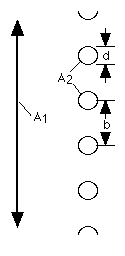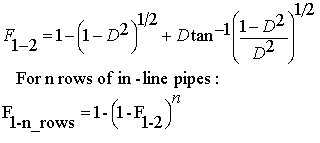Cody

# Problem 2831. Radiation Heat Transfer — View Factors (4)

Created by goc3 in Community

View factors (aka configuration factors) are utilized in some radiation heat transfer models to estimate heat transfer rates between surfaces. In particular, the thermal energy leaving a given surface is applied to other surfaces, as appropriate, based on how much the hot surface "sees" the other surfaces. As such, view factors are purely geometrical in nature. A range of view factor formulae are available here.

For this problem, calculate the view factor from surface 1 (an infinitely long plate) to surfaces 2 (n rows of in-line pipes):The view factor for one row of pipes is F_1-2 (the first equation):The second equation is utilized for more than one row of pipes. Any of the variables can be a vector. Also, note that D = d/b, where d is the pipe diameter and b is the center-to-center spacing between pipes.

### Solution Stats

48.39% Correct | 51.61% Incorrect
Last solution submitted on Jun 16, 2019